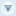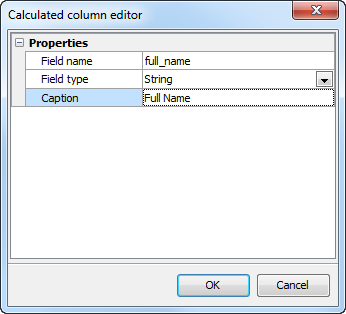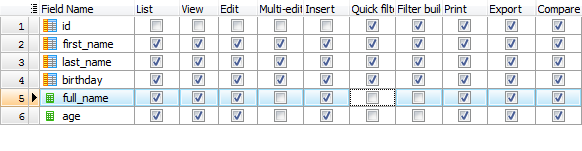Subscribe to our news:PartnersCustomers

Calculated ColumnsCalculated (AKA computed, generated, or virtual) columns are columns that do not exist in the data source the page is based on and their values are automatically computed using other column values or another deterministic expression.  For example, you might create a string column that displays concatenated values from other fields, create an integer column to calculate the person age based on his birthday, and so on.

Creating calculated columns

To create a new calculated column, open Page Editor, right-click the grid at the Columns tab and choose Add calculated column... from the popup menu. The following window will be opened:Provide the name, the data type and the caption for the new column and press OK. A new calculated column will be added to the column list. To quickly distinguish between ordinal and calculated fields, the latter are marked with a green calculator icon.It is possible to add multiple calculated columns for a single page.

Dropping calculated columns

To drop an existing calculated column, select the column in the column list, right-click and choose Drop calculated column from the popup menu.

Compute column values

To compute column values in read-only views (List, Print, Export, etc) provide the OnCalculateFields server-side event handler. For Edit and Insert forms provide the OnCalculateControlValues client-side event handler.

Use totals

Check this option to aggregate values this calculated column, specify the Aggregate expression (like SUM(quantity*price) where quantity and price are the table column names) and the Aggregate caption. Total content of calculated columns can be customized via OnCustomRenderTotals event.

Restrictions

Calculated columns cannot be used with Data Filtering and Multi-Edit tools, so the corresponding options in the column list are always disabled.